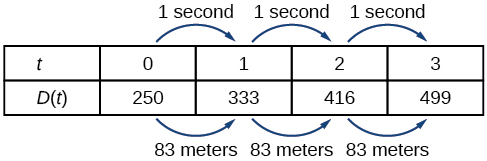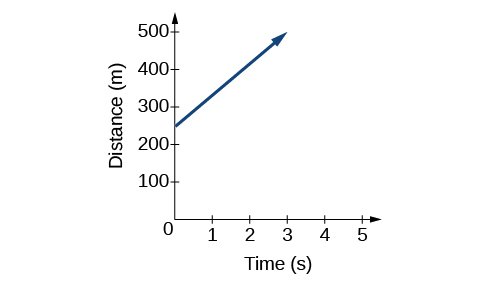2.1 Linear functions  (Page 2/17)

 Page 2 / 17
$D\left(t\right)=83t+250$

Representing a linear function in tabular form

A third method of representing a linear function is through the use of a table. The relationship between the distance from the station and the time is represented in [link] . From the table, we can see that the distance changes by 83 meters for every 1 second increase in time.Tabular representation of the function D showing selected input and output values

Can the input in the previous example be any real number?

No. The input represents time, so while nonnegative rational and irrational numbers are possible, negative real numbers are not possible for this example. The input consists of non-negative real numbers.

Representing a linear function in graphical form

Another way to represent linear functions is visually, using a graph. We can use the function relationship from above, $D\left(t\right)=83t+250,$ to draw a graph, represented in [link] . Notice the graph is a line. When we plot a linear function, the graph is always a line.

The rate of change, which is constant, determines the slant, or slope of the line. The point at which the input value is zero is the vertical intercept, or y -intercept , of the line. We can see from the graph in [link] that the y -intercept in the train example we just saw is $\left(0,250\right)$ and represents the distance of the train from the station when it began moving at a constant speed.The graph of D ( t ) = 83 t + 250. Graphs of linear functions are lines because the rate of change is constant.

Notice that the graph of the train example is restricted, but this is not always the case. Consider the graph of the line $f\left(x\right)=2{x}_{}+1.$ Ask yourself what numbers can be input to the function, that is, what is the domain of the function? The domain is comprised of all real numbers because any number may be doubled, and then have one added to the product.

Linear function

A linear function    is a function whose graph is a line. Linear functions can be written in the slope-intercept form of a line

$f\left(x\right)=mx+b$

where $b$ is the initial or starting value of the function (when input, $x=0$ ), and $m$ is the constant rate of change, or slope    of the function. The y -intercept is at $\left(0,b\right).$

Using a linear function to find the pressure on a diver

The pressure, $P,$ in pounds per square inch (PSI) on the diver in [link] depends upon her depth below the water surface, $d,$ in feet. This relationship may be modeled by the equation, $P\left(d\right)=0.434d+14.696.$ Restate this function in words.(credit: Ilse Reijs and Jan-Noud Hutten)

To restate the function in words, we need to describe each part of the equation. The pressure as a function of depth equals four hundred thirty-four thousandths times depth plus fourteen and six hundred ninety-six thousandths.

Determining whether a linear function is increasing, decreasing, or constant

The linear functions we used in the two previous examples increased over time, but not every linear function does. A linear function may be increasing, decreasing, or constant. For an increasing function    , as with the train example, the output values increase as the input values increase. The graph of an increasing function has a positive slope. A line with a positive slope slants upward from left to right as in [link] (a) . For a decreasing function    , the slope is negative. The output values decrease as the input values increase. A line with a negative slope slants downward from left to right as in [link] (b) . If the function is constant, the output values are the same for all input values so the slope is zero. A line with a slope of zero is horizontal as in [link] (c) .

find the equation of the line if m=3, and b=-2
graph the following linear equation using intercepts method. 2x+y=4
Ashley
how
Wargod
what?
John
ok, one moment
UriEl
how do I post your graph for you?
UriEl
it won't let me send an image?
UriEl
also for the first one... y=mx+b so.... y=3x-2
UriEl
y=mx+b you were already given the 'm' and 'b'. so.. y=3x-2
Tommy
"7"has an open circle and "10"has a filled in circle who can I have a set builder notation
x=-b+_Гb2-(4ac) ______________ 2a
I've run into this: x = r*cos(angle1 + angle2) Which expands to: x = r(cos(angle1)*cos(angle2) - sin(angle1)*sin(angle2)) The r value confuses me here, because distributing it makes: (r*cos(angle2))(cos(angle1) - (r*sin(angle2))(sin(angle1)) How does this make sense? Why does the r distribute once
so good
abdikarin
this is an identity when 2 adding two angles within a cosine. it's called the cosine sum formula. there is also a different formula when cosine has an angle minus another angle it's called the sum and difference formulas and they are under any list of trig identities
strategies to form the general term
carlmark
How can you tell what type of parent function a graph is ?
generally by how the graph looks and understanding what the base parent functions look like and perform on a graph
William
if you have a graphed line, you can have an idea by how the directions of the line turns, i.e. negative, positive, zero
William
y=x will obviously be a straight line with a zero slope
William
y=x^2 will have a parabolic line opening to positive infinity on both sides of the y axis vice versa with y=-x^2 you'll have both ends of the parabolic line pointing downward heading to negative infinity on both sides of the y axis
William
y=x will be a straight line, but it will have a slope of one. Remember, if y=1 then x=1, so for every unit you rise you move over positively one unit. To get a straight line with a slope of 0, set y=1 or any integer.
Aaron
yes, correction on my end, I meant slope of 1 instead of slope of 0
William
what is f(x)=
I don't understand
Joe
Typically a function 'f' will take 'x' as input, and produce 'y' as output. As 'f(x)=y'. According to Google, "The range of a function is the complete set of all possible resulting values of the dependent variable (y, usually), after we have substituted the domain."
Thomas
Sorry, I don't know where the "Â"s came from. They shouldn't be there. Just ignore them. :-)
Thomas
Darius
Thanks.
Thomas
Â
Thomas
It is the Â that should not be there. It doesn't seem to show if encloses in quotation marks. "Â" or 'Â' ... Â
Thomas
Now it shows, go figure?
Thomas
what is this?
i do not understand anything
unknown
lol...it gets better
Darius
I've been struggling so much through all of this. my final is in four weeks 😭
Tiffany
this book is an excellent resource! have you guys ever looked at the online tutoring? there's one that is called "That Tutor Guy" and he goes over a lot of the concepts
Darius
thank you I have heard of him. I should check him out.
Tiffany
is there any question in particular?
Joe
I have always struggled with math. I get lost really easy, if you have any advice for that, it would help tremendously.
Tiffany
Sure, are you in high school or college?
Darius
Hi, apologies for the delayed response. I'm in college.
Tiffany
how to solve polynomial using a calculator
So a horizontal compression by factor of 1/2 is the same as a horizontal stretch by a factor of 2, right?
The center is at (3,4) a focus is at (3,-1), and the lenght of the major axis is 26
The center is at (3,4) a focus is at (3,-1) and the lenght of the major axis is 26 what will be the answer?
Rima
I done know
Joe
What kind of answer is that😑?
Rima
I had just woken up when i got this message
Joe
Can you please help me. Tomorrow is the deadline of my assignment then I don't know how to solve that
Rima
i have a question.
Abdul
how do you find the real and complex roots of a polynomial?
Abdul
@abdul with delta maybe which is b(square)-4ac=result then the 1st root -b-radical delta over 2a and the 2nd root -b+radical delta over 2a. I am not sure if this was your question but check it up
Nare
This is the actual question: Find all roots(real and complex) of the polynomial f(x)=6x^3 + x^2 - 4x + 1
Abdul
@Nare please let me know if you can solve it.
Abdul
I have a question
juweeriya
hello guys I'm new here? will you happy with me
mustapha
The average annual population increase of a pack of wolves is 25.
how do you find the period of a sine graph
Period =2π if there is a coefficient (b), just divide the coefficient by 2π to get the new period
Am
if not then how would I find it from a graph
Imani
by looking at the graph, find the distance between two consecutive maximum points (the highest points of the wave). so if the top of one wave is at point A (1,2) and the next top of the wave is at point B (6,2), then the period is 5, the difference of the x-coordinates.
Am
you could also do it with two consecutive minimum points or x-intercepts
Am
I will try that thank u
Imani
Case of Equilateral Hyperbola
ok
Zander
ok
Shella
f(x)=4x+2, find f(3)
Benetta
f(3)=4(3)+2 f(3)=14
lamoussa
14
Vedant
pre calc teacher: "Plug in Plug in...smell's good" f(x)=14
Devante
8x=40
Chris
Explain why log a x is not defined for a < 0
the sum of any two linear polynomial is whatByByBy